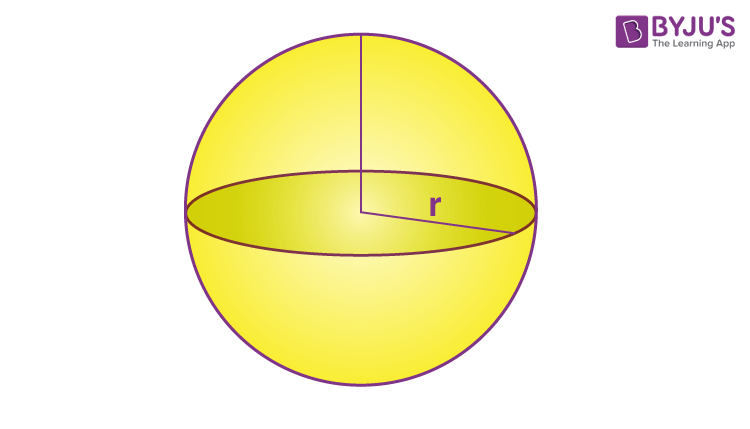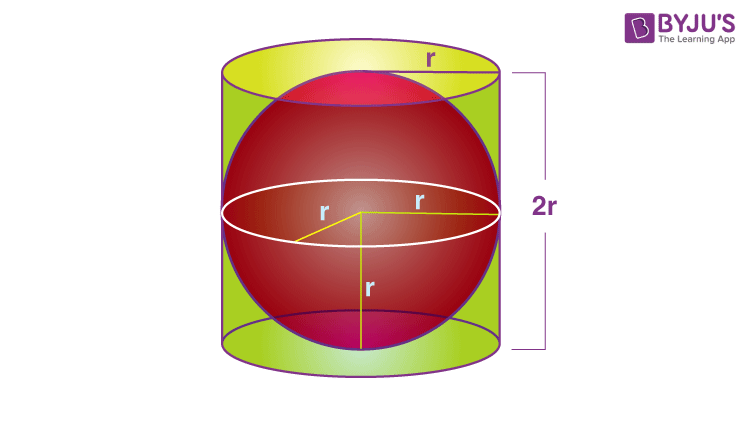Checkout JEE MAINS 2022 Question Paper Analysis : Checkout JEE MAINS 2022 Question Paper Analysis :

# Sphere

A sphere is a three-dimensional object that is round in shape. The sphere is defined in three axes, i.e., x-axis, y-axis and z-axis. This is the main difference between circle and sphere. A sphere does not have any edges or vertices, like other 3D shapes

The points on the surface of the sphere are equidistant from the center. Hence, the distance between the center and the surface of the sphere are equal at any point. This distance is called the radius of the sphere. Examples of spheres are a ball, a globe, the planets, etc.

## What is a Sphere?

As discussed in the introduction, the sphere is a geometrical figure that is round in shape. The sphere is defined in a three-dimensional space. The sphere is three dimensional solid, that has surface area and volume. Just like a circle, each point of the sphere is at an equal distance from the center.

 Radius The distance between surface and center of the sphere is called its radius Diameter The distance from one point to another point on the surface of the sphere, passing through the center, is called its diameter. Surface area The region occupied by the surface of the sphere is called it’s surface area Volume The amount of space occupied by any spherical object is called its volumeIn the above figure, we can see, a sphere with radius ‘r’.

Unlike a circle, which is a plane shape or flat shape, defined in XY plane, a sphere is defined in three dimensions, i.e. x-axis, y-axis and z-axis.

### Important Facts on Sphere

• A sphere is a symmetrical object
• All the surface points of the sphere are equidistant from center
• A sphere has an only a curved surface, no flat surface, no edges and no vertices

## Shape of Sphere

The shape of a sphere is round and it does not have any faces. The sphere is a geometrical three-dimensional solid having a curved surface. Like other solids, such as cube, cuboid, cone and cylinder, a sphere does not have any flat surface or a vertex or an edge.

The real-life examples of the sphere are:

• World Globe
• Marbles
• Planets
• Moon

## Properties of a sphere

The important properties of the sphere are given below. These properties are also called attributes of the sphere.

• A sphere is perfectly symmetrical
• A sphere is not a polyhedron
• All the points on the surface are equidistant from the center
• A sphere does not have a surface of centers
• A sphere has constant mean curvature
• A sphere has a constant width and circumference.

## Equation of a Sphere

In analytical geometry, if “r” is the radius, (x, y, z) is the locus of all points and (x0, y0, z0) is the center of a sphere, then the equation of a sphere is given by:

 (x -x0)2 + (y – y0)2 + (z-z0)2 = r2

## Sphere Formulas

The common formulas of the sphere are:

• Surface area
• Volume
 Diameter of sphere D = 2r, where r is the radius Surface area of sphere SA = 4πr2 Square units Volume of sphere V = 4/3 πr3 Cubic Units

### Surface Area of a Sphere

The surface area of a sphere is the total area covered by the surface of a sphere in a three-dimensional space. The formula of surface are is given by:

 The Surface Area of a Sphere(SA) = 4πr2 Square units

Where “r” is the radius of the sphere.

### Volume of a Sphere

The amount of space occupied by the object three-dimensional object called a sphere is known as the volume of the sphereAccording to the Archimedes Principle, the volume of a sphere is given as,

 The volume of Sphere(V) = 4/3 πr3 Cubic Units

## Difference Between a Sphere and a Circle

A circle and a sphere are shapes in geometry, that appear the same, but are different in properties. The key differences between the two shapes are listed below in the table.

 Circle Sphere A circle is a two-dimensional or 2d shape A sphere is a three-dimensional or 3d shape A circle is defined by two axes, the x-axis and the y-axis. A sphere is defined by three axes, x-axis, y-axis and z-axis The region occupied by a circle is simply an area. The formula of the area is πr2 A sphere has a surface area covered by its outer surface, which is equal to 4πr2 It does not have any volume It has volume It has a flat face It has no flat face but a curved face

## Solved Examples on Sphere

Example 1:

Find the volume of the sphere that has a diameter of 10 cm?

Solution:

Given, Diameter, d = 10 cm

We know that D = 2 r units

Therefore, the radius of a sphere, r = d / 2 = 10 / 2 = 5 cm

To find the volume:

The volume of sphere = 4/3 πr3 Cubic Units

V = (4/3)× (22/7) ×53

Therefore, the volume of sphere, V = 522 cubic units

Example 2:

Determine the surface area of a sphere having a radius of 7 cm.

Solution:

The Surface Area of a Sphere(SA) = 4πr2 Square units

SA = 4× (22/7)× 72

SA = 4 × 22 × 7

SA = 616 cm2

Therefore, the surface area of a sphere = 616 square units.

Example 3:

Find the volume of a sphere in terms of π, if the radius is 9 cm?

Solution:

Given: Radius, r = 9 cm.

We know that the volume of a sphere is 4/3 πr3 Cubic Units

Now, substitute r = 9 in the formula, we get

V = (4/3)× π × 9 × 9 × 9 cm3

V = 4 × π × 3 × 9 × 9 cm3

V = 972 π  cm3

Hence, the volume of a sphere is 972 cubic centimeters, if the radius is 9 cm.

### Practice Questions

1. Find the volume of the sphere if diameter = 10cm.
2. If the radius of a sphere is 14 cm, then find its surface area.
3. A cricket ball with radius ‘r’ cm and a basketball with radius ‘4r’ have volume in the ratio of?
4. Metallic spheres of radii 3 cm, 4 cm and 5 cm, respectively, are melted to form a single solid sphere. Find the radius of the resulting sphere.

Register with BYJU’S – The Learning App to learn about other three-dimensional shapes also watch interactive videos to learn with ease.

## Frequently Asked Questions on Sphere

### What is a sphere?

A sphere is three dimensional, geometrical shape, that has all its surface points equidistant from a common point. The distance between the surface and the common point is the radius and the common point is called center of sphere.

### How many sides does a sphere have?

A sphere does not have any sides, since it is a round-shaped object. It has a curved surface and not a flat surface.

### Is a sphere, circle?

A circle is a two dimensional shape, that has area and perimeter only. A sphere is a three dimensional shape, that has surface area and volume.

### What is Hemisphere?

A hemisphere is exactly half of a sphere. It has a curved surface and a flat surface.

### What are the characteristics of a sphere?

A sphere is symmetrical, round in shape. It is a three dimensional solid, that has all its surface points at equal distances from the center. It has surface area and volume based on its radius. It does not have any faces, corners or edges.

### What are the examples of spheres?

Football, Basketball, Globe, Planets, etc. are examples of sphere.

### What is surface area and volume of sphere?

The surface area of a sphere is the total area covered by surface of a sphere in three-dimension space. The formula for surface area is:
SA = 4πr2 Square units
Volume of sphere is the space occupied by sphere in three dimension space. The formula is:
V = 4/3πr3